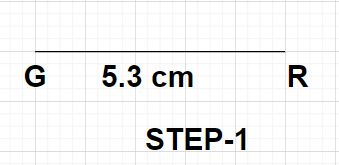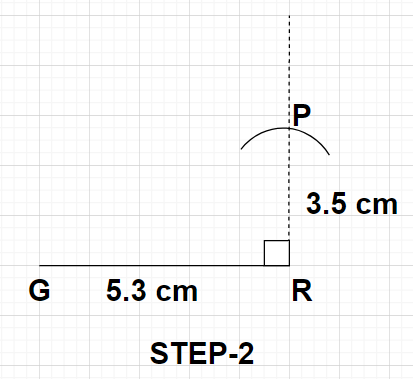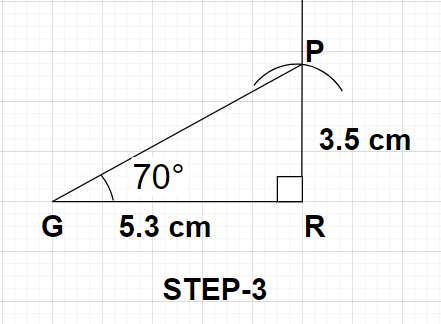Courses
Courses for Kids
Free study material
Free LIVE classes
More# Construct $\vartriangle {\text{GPR}}$ such that GR = 5.3 cm, $\angle {\text{GPR}} = {70^0}$, ${\text{PR}} \bot {\text{GR}}$ and PR = 3.5 cm.Verified
325.8k+ views
Hint: Here, we will proceed by applying the basic principles of construction and make the use of a scale and compass for the construction purpose and for verification we will use a protector.

Step 1- Firstly, take the scale and draw a line GR having a length of 5.3 cm as shown in the figure.Step 2- Through the point R, draw a perpendicular and then cut an arc of length 3.5 cm using the compass and the point where this arc meets the perpendicular mark that point as P as shown in the figure.Step 3- Now, join the points G and P with the help of the scale. This will result in the construction of the required triangle GPR as shown in the figure. We can also verify whether the triangle constructed is correct or not by measuring the angle $\angle {\text{GPR}}$ with the help of the protector. When this angle is measured it gives a value of ${70^0}$ which is true (given).Note: In this particular problem, an additional input data i.e., $\angle {\text{GPR}} = {70^0}$ is given which is not required for the construction of the triangle GPR but can be used for verification. If $\angle {\text{GPR}}$ gives a measure equal to ${70^0}$ then the constructed triangle is correctly constructed, else some mistake is being done.
Last updated date: 26th May 2023
Total views: 325.8k
Views today: 4.85k